• 一、对图像文件分别进行灰度线性变换、直方图、直方图均衡化操作 rgb=imread(‘lena.bmp’); gray1=rgb2gray(rgb); % 色彩转换成灰度 a=0.5; b=150; % 可根据实际需要进行改变 gray2=a*gray1+b; figure(1) ...
一、对图像文件分别进行灰度线性变换、直方图、直方图均衡化操作
rgb=imread(‘lena.bmp’);
gray1=rgb2gray(rgb); % 色彩转换成灰度
a=0.5; b=150; % 可根据实际需要进行改变
gray2=a*gray1+b;
figure(1)
subplot(1,2,1),imshow(gray1,[]),title(‘原图’);
subplot(1,2,2),imshow(gray2,[]),title(‘线性变换’);
figure(2)
subplot(3,2,1);  imshow(gray1); title(‘原图’);
subplot(3,2,2);  imhist(gray1); title(‘原图直方图’);
subplot(3,2,3);
H1=adapthisteq(gray1);
imshow(H1); title(‘adapthisteq均衡后图’);
subplot(3,2,4);
imhist(H1);title(‘adapthisteq均衡后直方图’);
subplot(3,2,5);
H2=histeq(gray1);
imshow(H2); title(‘histeq均衡后图’);
subplot(3,2,6);
imhist(H1); title(‘histeq均衡后直方图’);
二、对图像文件分别进行平移、垂直镜像变换、水平镜像变换、缩放和旋转操作。
clc
clear all
close all
A=imread(‘lena.bmp’);
[height,width,dim]=size(A);
tform=maketform(‘affine’,[-1 0 0; 0 1 0;width 0 1]); %定义水平镜像变换矩阵
B=imtransform(A,tform,‘nearest’);%%%‘nearest’：插值方式
tform2=maketform(‘affine’,[1 0 0; 0 -1 0;0 height 1]); %定义竖直镜像变换矩阵
C=imtransform(A,tform2,‘nearest’);
figure(2)
subplot(1,3,1),imshow(A),title(‘原图’);
subplot(1,3,2),imshow(B),title(‘水平镜像’);
subplot(1,3,3),imshow©,title(‘垂直镜像’);
j=imrotate(A,30);%图像旋转30度
k=imresize(A,2);%图像放大两倍
t=imresize(A,2,‘bilinear’);%采用双线性插值法进行放大两倍
m=imresize(A,0.8);%图像缩小到0.8倍
p=translate(strel(1),[25 25]);%图像平移
img=imdilate(A,p);
figure(3);
subplot(2,3,1),
imshow(A);
title(‘原图’);
subplot(2,3,2),
imshow(j);
title(‘旋转’);
subplot(2,3,3)
imshow(k);
title(‘放大’);
subplot(2,3,4)
imshow(t);
title(‘双线性插值’);
subplot(2,3,5)
imshow(m);
title(‘缩小’);
figure(1);
imshow(img);
title(‘平移’);
三、1、对图像文件分别进行均值滤波、中值滤波和拉普拉斯锐化操作；添加噪声，重复上述过程观察处理结果。
2、编写代码完成罗伯特算子、soble算子等不同的图像锐化，对其进行比较
clc
A1=imread(‘lena.bmp’); %读入图像
A=rgb2gray(A1);
%平均滤波（滑动窗口）
b1=fspecial(‘average’,3);%生成滤波算子
B1=imfilter(A,b1);
B2=medfilt2(A); %中值滤波
AA=double(A);
w1=[0 1 0; 1 -4 1; 0 1 0];
B3=imfilter(AA,w1,‘corr’,‘replicate’); %拉普拉斯锐化滤波，在滤波过程中使用相关；图像大小通过复制外边界的值来扩展，以此来处理边界充零问题
AAA=imnoise(A,‘salt & pepper’,0.25); %加噪声
C1=imfilter(AAA,b1);
C2=medfilt2(AAA);
C3=imfilter(double(AAA),w1,‘corr’,‘replicate’);
figure(1),
subplot(2,5,1),imshow(A),title(‘原图’);
subplot(2,5,2),imshow(B1),title(‘均值滤波’);
subplot(2,5,3),imshow(B2),title(‘中值滤波’);
subplot(2,5,4),imshow(B3,[]),title(‘拉普斯锐化’);
subplot(2,5,5),imshow(AA-B3,[]),title(‘锐化后图像’);
subplot(2,5,6),imshow(AAA),title(‘加噪声’);
subplot(2,5,7),imshow(C1),title(‘均值滤波’);
subplot(2,5,8),imshow(C2),title(‘中值滤波’);
subplot(2,5,9),imshow(C3,[]),title(‘拉普斯锐化’);
A2=A1;
A3=A1;
[m,n]=size(A1);
figure,imshow(A1);
A1=double(A1);
b=zeros(m,n);
c=zeros(m,n);
for i=1:m-2
for j=1:n-2
b(i+1,j+1)=A1(i,j)-A1(i+1,j+1);
c(i+1,j+1)=A1(i,j+1)-A1(i+1,j);
b(i+1,j+1)=sqrt(b(i+1,j+1)2+c(i+1,j+1)2)+100;
end
end
figure(2);imshow(uint8(b)),title(‘Roberts’);
[m,n]=size(A2);
figure,imshow(A2);
A2=double(A2);
b=zeros(m,n);
c=zeros(m,n);
for i=1:m-2
for j=1:n-2
b(i+1,j+1)=-A2(i,j)-A2(i+1,j)-A2(i+2,j+2)+A2(i,j+2)+A2(i+1,j+2)+A2(i+2,j+2);
c(i+1,j+1)=A2(i,j)+A2(i,j+1)+A2(i,j+2)-A2(i+2,j)-A2(i+2,j+1)-A2(i+2,j+2);
b(i+1,j+1)=sqrt(b(i+1,j+1)2+c(i+1,j+1)2)+100;
%这儿处理有两种方式，绝对值和统一加一个数,不同的处理会得到不同的效果
%if b(i+1,j+1)<0
% b(i+1,j+1)=-b(i+1,j+1);
%end
end
end
figure(3);imshow(uint8(b)),title(‘priwitt’);
[m,n] = size(A3); % 得到图像的大小
new_img_gray = A3; % 新建一个一样大的图像
pxValue = 0; % roberts计算所得到的像素值
% 对边界象素操作
for threshold_value=5:5:90
for i=1:m-1
for j=1:n-1
pxValue = abs(A3(i,j)-A3(i+1,j+1))+…
abs(A3(i+1,j)-A3(i,j+1));
if(pxValue > threshold_value)
new_img_gray(i,j) = 255;
else
new_img_gray(i,j) = 0;
end
end
end
figure(4)
subplot(3,6,threshold_value/5);
imshow(new_img_gray);
title([‘soble’,num2str(threshold_value)])
end
四、启动MATLAB程序，对图像文件分别进行二维Fourier变换，将其幅度谱进行搬移，在图像中心显示，并评价人眼对图像幅频特性和相频特性的敏感度。
编写代码实现由频谱重建原图像、幅度谱重建图像和相位谱重建图像
I=imread(‘lena.bmp’);
I1=rgb2gray(I);
fftI=fft2(I1);%二维离散傅立叶变换
sfftI=fftshift(fftI);%直流分量移到频谱中心
RR=real(sfftI);%取傅立叶变换的实部
II=imag(sfftI); %取傅立叶变换的虚部
A=sqrt(RR.2+II.2); %计算频谱幅值
B=(A-min(min(A)))./(max(max(A))-min(min(A)))*225; %归一化
phA=log(angle(sfftI)180/pi);
figure(1)%设定窗口
subplot(2,2,1),imshow(I1);title(‘原图’);
subplot(2,2,2),imshow(B);title(‘原图像的频谱’); %显示原图像的频谱
subplot(2,2,3),imshow(log(B),[]);title(‘原图像的幅度’); %显示原图像的幅度
subplot(2,2,4),imshow(phA);title(‘原图的相位谱’);
W=abs(ifft2(abs(sfftI).exp(1j(angle(sfftI)))));
w=abs(ifft2(abs(sfftI)));%幅度重构
Q=abs(ifft2(exp(1j(angle(sfftI)))));
figure(2)
subplot(131),imshow(W,[]);
subplot(132),imshow(w,[]);
subplot(133),imshow(Q,[]);
五、1、编写代码观察频率拉普拉斯滤波器
2、编写代码实现高斯低通滤波器对图像进行处理
3、编写代码实现高斯高通滤波器对图像进行处理
clear
clc
close all
f=imread(‘lena.bmp’);
f=rgb2gray(f);
subplot(1,3,1);imshow(f);title(‘原始图像’)
F=fft2(f);             %对图像进行傅立叶变换
F1=fftshift(F);
%对图像进行高斯高通滤波
[M,N]=size(f);
sigma=20;
K=0;
for i=1:M
for j=1:N
g(i,j) = 1 - exp (-(( i-M/2)^ 2+( j-N/2)^ 2)/ 2/ sigma^2)+K;
end
end
figure(1)
subplot(1,3,2);imshow(g);title(‘高斯高通滤波’)
GF=F.*g;
gf=ifft2(GF);
subplot(1,3,3);imshow(gf,[]);title(‘高斯高通滤波图像’)
mask=[0,1,0;1,-4,1;0,1,0];%拉普拉斯滤波模板
C=imfilter(f,mask,‘replicate’);
figure(2),imshow©;title(‘拉普拉斯滤波器’);
d=10
for i=1:M;
for j=1:N
distance=sqrt((i-M/2)2+(j-N/2)2);
if distance<=d
flag=1;
else
flag=0;
end
LF(i,j)=flag*F1(i,j);
end
end
%逆变换转换成对应图像
LF=uint8(real(ifft2(ifftshift(LF))));
figure(3),imshow(LF);
title(‘低通滤波后图像’);
六、1、逆滤波
2、编写代码实现图像的模糊、加噪和维纳滤波复原。
clear
clc
close all
I0=imread(‘456.jpg’);
I0=imresize(rgb2gray(I0),0.25);
figure(1)
subplot(1,3,1);
imshow(I0);title(‘原始图像’);
I=double(I0);
[m,n]=size(I);
F=fftshift(fft2(I));
k=0.00025;
for u=1:m
for v=1:n;
H(u,v)=exp((-k)*(((u-m/2)2+(v-n/2)2)^(5/6)));
end
end
F1=F./H;
I2=ifft2(fftshift(F1));
subplot(1,3,2);
imshow(uint8(I2));title(‘全逆滤波复原图像’);
BanJing=50;
H=ones(size(I0));
for u=(m/2-BanJing):(m/2+BanJing)
for v=(n/2-BanJing):(n/2+BanJing)
H(u,v)=exp((-k)*(((u-m/2)2+(v-n/2)2)^(5/6)));
end
end
F1=F./H;
I2=ifft2(fftshift(F1));
subplot(1,3,3);
imshow(uint8(I2));title(‘限制逆滤波复原图像’);
PSF = fspecial(‘motion’,30,25);
I1= imfilter(I0,PSF,‘conv’,‘circular’);
noise = 0.1*randn(size(I0));%设定一个高斯噪声
Noise = imadd(I1,im2uint8(noise));%对模糊后图像加噪
R1=wiener2(Noise,[10 10]); %未知噪声
R2=wiener2(Noise,[10 10],noise);%已知噪声分布
figure(2)
subplot(2,3,1),imshow(uint8(I0));title(‘原始图像’);
subplot(2,3,2),imshow(uint8(I1));title(‘模糊后图像’);
subplot(2,3,3),imshow(uint8(Noise));title(‘加噪后图像’);
subplot(2,3,4),imshow(uint8(R1));title(‘盲复原’);
subplot(2,3,5),imshow(uint8(R2));title(‘非盲复原’);
七、1）腐蚀、膨胀开闭运算
2）编写代码实现二值图像区域填充：
3）编写代码实现边缘轮廓提取程序：
% rgb=imread(‘source1.bmp’);
% I=rgb2gray(rgb); % 色彩转换成灰度
I=imread(‘source3.bmp’);
level= graythresh(I);  %得到合适的阈值 得到合适的阈值
bw= im2bw(I,level);  %二值化
SE= strel(‘square’,3); %设置膨胀结构元素
BW1= imdilate(bw,SE);            %    膨胀
SE1= strel(‘arbitrary’,eye(5));       %设置腐蚀结构元素
BW2= imerode(bw,SE1);                 %腐蚀
BW3= bwmorph(bw,‘open’);              %开运算
BW4= bwmorph(bw,‘close’);             %闭运算
figure(1),
subplot(2,3,1),imshow(I);title(‘原图’);
subplot(2,3,2),imshow(bw);title(‘二值图’);
subplot(2,3,3),imshow(BW1);title(‘膨胀’);
subplot(2,3,4),imshow(BW2);title(‘腐蚀’);
subplot(2,3,5),imshow(BW3);title(‘开运算’);
subplot(2,3,6),imshow(BW4);title(‘闭运算’);
im=imfill(bw,‘holes’);
im1=bwperim(bw);
figure(2)
subplot(121),imshow(im,[]);title(‘二值图填充图’)
subplot(122),imshow(im1,[]);title(‘轮廓提取图’)
八、1）使用Canny、Roberts、Sobel、Prewitt、LoG等算子对灰度图像进行边缘检测：
2)编写代码实现双峰法图像分割
3)编写代码实现Otsu法图像分割
clc
I=double(imread(‘source3.bmp’)); %读入图像
I1 = imread(‘source3.bmp’);
im_median=medfilt2(I);
BW1=edge(I,‘roberts’); % roberts算子
BW2=edge(I,‘prewitt’);
BW3=edge(I,‘sobel’);
BW4=edge(I,‘log’);
BW5= edge(I,‘canny’);
figure(1),
subplot(231),imshow(I,[]);title(‘原图’);
subplot(232),imshow(BW1); title(’ roberts算子’);
subplot(233),imshow(BW2); title(’ prewitt算子’);
subplot(234),imshow(BW3); title(’ sobel算子’);
subplot(235),imshow(BW4); title(’ log算子’);
subplot(236),imshow(BW5); title(’ canny算子’);
if ndims(I1) == 3
I1 = rgb2gray(I1);
end
fxy = imhist(I1, 256); %统计每个灰度值的个数
figure(2);
subplot(2, 2, 1); imshow(I1, []); title(‘原图’)
subplot(2, 2, 2); plot(fxy); %画出灰度直方图
title(‘直方图’)
p = 168;
p = p/255;
bw = im2bw(I1, p); %小于阈值的为黑，大于阈值的为白
subplot(2, 2, 3); imshow(bw); title(‘双峰阈值分割’)
bw1 = im2bw(I1, graythresh(I1));
subplot(2, 2, 4); imshow(bw1); title(‘ostu阈值分割’)


展开全文• 自动点焊技术之数字图像处理 实验报告 实验名称:直方图均衡化增强 实验编号:Po03-02 作 者珠海博泰科技莫工 学 号04 实验日期:2014年11月0日 摘 要本实验通过计算原始图像以及直方图均衡化后图像的直方图,验证书本中...
• 自动点焊技术之数字图像处理 实验报告 实验名称直方图均衡化增强 实验编号Proj03-02 作 者珠海博泰科技莫工 学 号20144309018 实验日期2014 年 11 月 30 日 摘 要本实验通过计算原始图像以及直方图均衡化后图像的...
• 图像处理MATLAB实现;一读取图像 使用函数imread可以将图像读入MATLAB 环境其语法格式为 imread'filename) 其中filename是一个含有图像文件全名字 符串 ; 当filename中不包含任何路径信息时imread会从当前目录中寻找...
• 数字图像处理 实验指导书 1 目录 实验MATLAB 数字图像处理初步 实验二 图像的代数运算 实验三 图像增强-空间滤波 实验四 图像分割 1 2 实验MATLAB 数字图像处理初步 一实验目的与要求 1熟悉及掌握在MATLAB 中...
• 数字图像处理 matlab实验 北邮 大四 包含三次实验报告和源代码
• 数字图像处理实验matlab程序代码数字图像处理实验数字图像处理实验PAGEPAGE 2数字图像处理实验matlab程序代码目录TOC \o "1-3" \h \z \u HYPERLINK \l "_Toc329720793" 实验MATLAB数字图像处理初步 PAGEREF _...
数字图像处理实验和matlab程序代码数字图像处理实验数字图像处理实验PAGEPAGE  2数字图像处理实验和matlab程序代码目录TOC \o "1-3" \h \z \u  HYPERLINK \l "_Toc329720793" 实验一  MATLAB数字图像处理初步 PAGEREF _Toc329720793 \h 2HYPERLINK \l "_Toc329720794" 实验二  图像的代数运算 PAGEREF _Toc329720794 \h 6HYPERLINK \l "_Toc329720795" 实验三  图像增强—灰度变换 PAGEREF _Toc329720795 \h 9HYPERLINK \l "_Toc329720796" 实验四  图像增强—直方图变换 PAGEREF _Toc329720796 \h 11HYPERLINK \l "_Toc329720797" 实验五  图像增强—空域滤波 PAGEREF _Toc329720797 \h 13HYPERLINK \l "_Toc329720798" 实验六  图像的傅立叶变换 PAGEREF _Toc329720798 \h 17HYPERLINK \l "_Toc329720799" 实验七  图像增强—频域滤波 PAGEREF _Toc329720799 \h 19HYPERLINK \l "_Toc329720800" 实验八  彩色图像处理 PAGEREF _Toc329720800 \h 21HYPERLINK \l "_Toc329720801" 实验九  图像分割 PAGEREF _Toc329720801 \h 24HYPERLINK \l "_Toc329720802" 实验十  形态学运算 PAGEREF _Toc329720802 \h 27实验一  MATLAB数字图像处理初步一、实验目的与要求1．熟悉及掌握在MATLAB中能够处理哪些格式图像。2．熟练掌握在MATLAB中如何读取图像。3．掌握如何利用MATLAB来获取图像的大小、颜色、高度、宽度等等相关信息。4．掌握如何在MATLAB中按照指定要求存储一幅图像的方法。5．图像间如何转化。二、实验内容及步骤1．利用imread( )函数读取一幅图像，假设其名为flower.tif，存入一个数组中；2．利用whos 命令提取该读入图像flower.tif的基本信息；3．利用imshow()函数来显示这幅图像；4．利用imfinfo函数来获取图像文件的压缩，颜色等等其他的详细信息；5．利用imwrite()函数来压缩这幅图象，将其保存为一幅压缩了像素的jpg文件,设为flower.jpg；语法：imwrite(原图像，新图像，‘quality’,q), q取0-100。6．同样利用imwrite()函数将最初读入的tif图象另存为一幅bmp图像，设为flower.bmp。7．用imread()读入图像：Lenna.jpg 和camema.jpg；8．用imfinfo()获取图像Lenna.jpg和camema.jpg 的大小；9．用figure,imshow()分别将Lenna.jpg和camema.jpg显示出来，观察两幅图像的质量。10．用im2bw将一幅灰度图像转化为二值图像，并且用imshow显示出来观察图像的特征。11．将每一步的函数执行语句拷贝下来，写入实验报告，并且将得到第3、9、10步得到的图像效果拷贝下来三、考核要点1、熟悉在MATLAB中如何读入图像、如何获取图像文件的相关信息、如何显示图像及保存图像等，熟悉相关的处理函数。2、明确不同的图像文件格式，由于其具体的图像存储方式不同，所以文件的大小不同，因此当对同一幅图像来说，有相同的文件大小时，质量不同。五、实验仪器与软件(1) PC计算机(2) MatLab软件/语言包括图像处理工具箱(Image Processing Toolbox)(3) 实验所需要的图片四、实验内容f=imread('C:\Users\Gateway\Desktop\í???\flower.tif');whos f;imshow(f);imfinfo C:\Users\Gateway\Desktop\í???\flower.tifans =Filename: 'C:\Users\Gateway\Desktop\图片\flower.tif'FileModDate: '24-六月-2012 18:46:53'FileSize: 392018
展开全文• 数字图像处理matlab实验中的一个，形态学方法实现边缘检测
• 数字图像处理Matlab-形态学图像处理 数学形态学 数字图像处理Matlab-图像压缩与离散余弦变换 数字图像处理Matlab-小波变换在图像处理中的应用 数字图像处理Matlab-彩色图像处理 数字图像处理Matlab-图像的滤波处理与...
本文旨在记录自己的一门非常用心学习的课程作业，以及和大家分享研究成果，喜欢的别忘了点一个赞哦~~~
数字图像处理–基于Matlab的人脸检测研究
数字图像处理Matlab-形态学图像处理
数学形态学
数字图像处理Matlab-图像压缩与离散余弦变换
数字图像处理Matlab-小波变换在图像处理中的应用
数字图像处理Matlab-彩色图像处理
数字图像处理Matlab-图像的滤波处理与图像空间变换
数字图像处理Matlab-傅里叶变换及其应用
数字图像处理Matlab-灰度直方图及直方图均衡化
数字图像处理Matlab-图像的显示与格式变换
码字不易，喜欢的不如点个赞哦~
我还写了很多文章，欢迎关注我哦~亲爱的朋友，这里是我的公众号，欢迎关注！
本博客的优秀博文也将陆续搬运到公众号，之后还将推出更多优秀博文，并将优先发在公众号，敬请期待！
关注起来，让我们一起成长！展开全文计算机视觉
• 数字图像处理matlab实验二，图像的代数运算。1．了解图像的算术运算在数字图像处理中的初步应用。 2．体会图像算术运算处理的过程和处理前后图像的变化。
• 实验MATLAB 数字图像处理初步 一实验目的与要求 熟悉及掌握在 MATLAB 中能够处理哪些格式图像 熟练掌握在 MATLAB 中如何读取图像 掌握如何利用 MATLAB 来获取图像的大小颜色高度宽度等等相关信息 掌握如何在 ...
• 前言：作为一名图像算法攻城狮，那是在2014年大三...通过本实验使学生掌握用MATLAB进行数字图像处理的基本语句。 二、实验原理 本实验室基于数字图像处理课程中的数字图像基本概念来设计的。 本实验的准备知识：MATLAB
前言：作为一名图像算法攻城狮，那是在2014年大三下学期，一本深绿色的《数字图像处理》（冈萨雷斯的英文版）出现在自己课桌前。偶然间打开的一扇意外之门，就这样结下了一段不解之缘，那些日子不断上网搜代码的自己，那个刚上机不到二十分钟就把作业提交的自己，早已随往日的岁月飘扬而去。三年的烟酒僧，两年的酱油工，而今只觉脑子越来越不够用，这次决心回炉重造，希望能够通过固本培基，打开思路，话不多说，开始上课！
----2020-7-6

实验1    数字图像处理的MATLAB基础
一、实验目的
通过本实验使学生掌握用MATLAB进行数字图像处理的基本语句。
二、实验原理
本实验室基于数字图像处理课程中的数字图像基本概念来设计的。
本实验的准备知识：MATLAB语言基础；数字图像的基本概念。其中会涉及到的命令包括MATLAB基本语句和图像处理工具箱内的函数。
三、实验内容
（一）复习MATLAB基本命令、基本绘图方法和编程方法
1、
A =
7     1     5
2     5     6
3     1     5
B =
1     1     1
2     2     2
3     3     3

A(2,3)
ans =
6

A(:,2)
ans =
1
5
1

A(3,:)
ans =
3     1     5

A(:,1:2:3)
ans =
7     5
2     6
3     5

A(:,3).*B(:,2)
ans =
5
12
15

A(:,3)*B(2,:)
ans =
10    10    10
12    12    12
10    10    10

A*B
ans =
24    24    24
30    30    30
20    20    20

A.*B
ans =
7     1     5
4    10    12
9     3    15

A^2
ans =
66    17    66
42    33    70
38    13    46

A.^2
ans =
49     1    25
4    25    36
9     1    25

B/A
ans =
0.1842    0.2105   -0.2368
0.3684    0.4211   -0.4737
0.5526    0.6316   -0.7105

B./A
ans =
0.1429    1.0000    0.2000
1.0000    0.4000    0.3333
1.0000    3.0000    0.6000

2、同屏绘制正弦曲线y=sin（x）、余弦曲线y=cos（x）、y=sin（x）cos(x)、y=sin(x)+cos(x)的图像。
(1)
x=0:0.01:2*pi;
y1=sin(x);
y2=cos(x);
y3=sin(x).*cos(x);
y4=sin(x)+cos(x);
plot(x,y1,x,y2,x,y3,x,y4);

运行结果：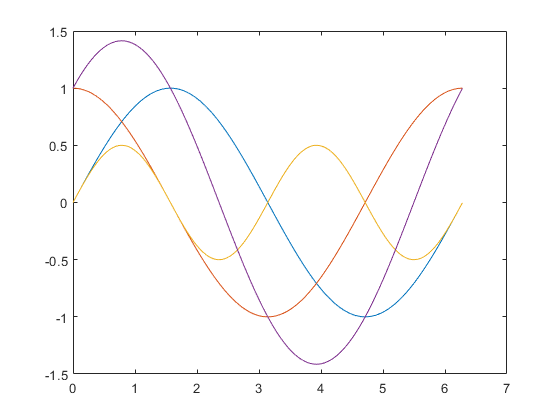(2)
x=-2:0.1:22;
y=x;
[X,Y]=meshgrid(x,y);
Z=X.^2*exp(-X.^2-Y.^2);
mesh(Z);

运行结果：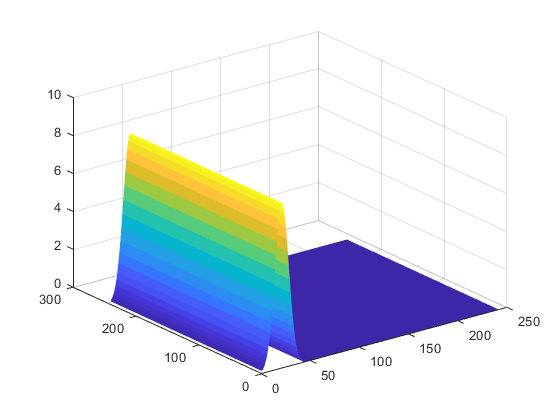3、
x=-2:0.1:2
y=-2:0.1:2
[X,Y]=meshgrid
Z=X.^2.*exp(-X.^2-Y.^2)
Mesh(Z)

运行结果：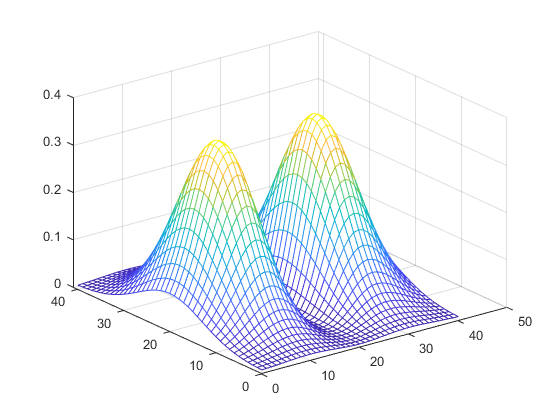4、编程计算30！

factorial(30)
ans =
2.6525e+032

**（二） 熟悉imread函数，imshow函数，imwrite函数的使用方法
1、 读入图像lena_gray.tif，并显示。读入图像rice.tif，并显示。
2、 令I=imread(‘lena_gray.tif’);求得图像的尺寸。显示图像I(20:200,20:100)，
将该图像存储为bmp 格式的图像。
3、 对图像rice.tif 和lena_gray.tif 进行加法、和旋转运算，并显示结果。
4、 读入彩色图像lena_color.tif，并显示。
5、实验程序及结果 ：
（1）	图像显示**
I=imread('F:\Images\lena.bmp');
figure;
subplot(2,2,1);
imshow(I,[20 200])
A=imread('F:\Images\rice.png');
subplot(2,2,2);
imshow(A,[20 200])
I=imread('F:\Images\lena.bmp');
subplot(2,2,3);
imshow(I,[20 100])
A=imread('F:\Images\rice.png');
subplot(2,2,4);
imshow(A,[20 100])

运行结果：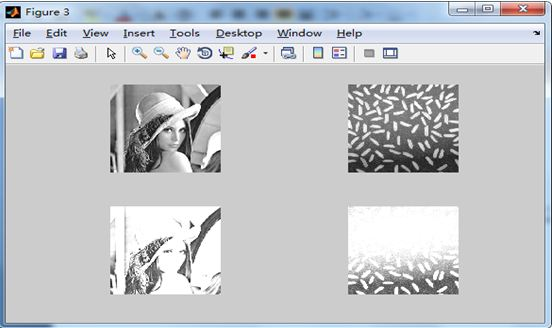（2）读入彩色图像
I=imread('F:\Images\lenacolor.BMP');
imshow(I);

运行结果：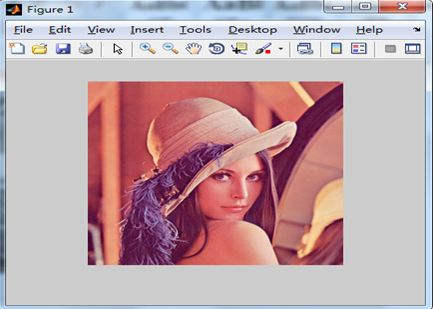展开全文矩阵 经验分享 计算机视觉
• 前言：作为一名图像算法攻城狮，那是在2014年大三下学期，一本绿色版的《数字图像处理》（冈萨雷斯的英文版）出现在自己课桌前。偶然间打开的一扇意外之门，就这样结下了一段不解之缘，那些...本实验是基于数字图像处理计算机视觉 课堂作业
• 前言：作为一名图像算法攻城狮，那是在2014年大三下学期，一本绿色版的《数字图像处理》（冈萨雷斯的英文版）出现在自己课桌前。偶然间打开的一扇意外之门...本实验是基于数字图像处理课程中的第六章-彩色图像处理理论计算机视觉 课堂作业
• * 一读取图像 使用函数imread可以将图像读入MATLAB 环境其语法格式为 imread'filename) 其中filename是一个含有图像文件全名的字 符串 常用图像操作 * 当filename中不包含任何路径信息时imread会从当前目录中寻找并...
• 前言：作为一名图像算法攻城狮，那是在2014年大三下学期，一本绿色版的《数字图像处理》（冈萨雷斯的英文版）出现在自己课桌前。偶然间打开的一扇意外之门，就这样结...本实验是基于数字图像处理课程中的图像变换和增强计算机视觉 课堂作业
• 前言：作为一名图像算法攻城狮，那是在2014年大三下学期，一本绿色版的《数字图像处理》（冈萨雷斯的英文版）出现在自己课桌前。偶然间打开的一扇意外之门，就这样结下...本实验是基于数字图像处理课程中的二维傅里叶变计算机视觉 课堂作业
• 前言：作为一名图像算法攻城狮，那是在2014年大三下学期，一本绿色版的《数字图像处理》（冈萨雷斯的英文版）出现在自己课桌前...本实验是基于数字图像处理课程中的图像频域增强理论来设计的。 本实验的准备知识：第四计算机视觉 课堂作业
• 数字图像处理实验 matlab 计算机图像处理 姓名 学号 班级 实验一了解数字图像处理平台 一实验目的 1学会使用扫描仪 2熟悉 MATLAB软件 二实验内容 1用扫描仪扫一幅彩色图片一幅灰度图片 2熟悉 MATLAB的主界面窗口中...
• MATLAB全称是Matrix Laboratory矩阵实验室一开始它是一种专门用于矩阵数值计算的软件从这一点上也可以看出它在矩阵运算上有自己独特的特点实际上MATLAB中的绝大多数的运算都是通过矩阵这一形式进行的这一特点也就...
• 前言：作为一名图像算法攻城狮，那是在2014年大三下学期，一本绿色版的《数字图像处理》（冈萨雷斯的英文版）出现在自己课桌前。偶然间打开的一扇意外之门，就...本实验是基于数字图像处理课程中的图像分割理论来设计计算机视觉 课堂作业
• 前言：作为一名图像算法攻城狮，那是在2014年大三下学期，一本绿色版的《数字图像处理》（冈萨雷斯...本实验是基于数字图像处理课程中的第五章图像复原理论来设计的。 本实验的准备知识：第五章图像复原。 重点如下： 1计算机视觉 课堂作业
• 前言：作为一名图像算法攻城狮，那是在2014年大三下学期，一本绿色版的《数字图像处理》（冈萨雷斯的英文版）出现在自己课桌前。偶然间打开的一扇意外之门，就这样结下了一段不解之缘，那些日子不断上网搜代码的自己...计算机视觉 课堂作业
• 实验一 内容一 1彩色图像变灰度图像 A=imread'1.jpg; B=rgb2gray(A; figure subplot(1,2,1, imshow(A) title'原图) subplot(1,2,2, imshow(B) title'原图灰度图像) 2彩色图像变索引图像 A=imread'1.jpg; figure ...
• 数字图像处理课程实验MATLAB程序实现，包括：灰度退、均衡化，边缘检测、灰度直方图、图像增强、伪彩色、DCT变换等
• 初学数字图像处理实验报告以及程序，先频域处理（陷波滤波）再空域（中值） 陷波采用的是设置全1矩阵，逐点处理的方法，下面是实验报告的描述，天鹅图像可以完全去除，小狗图像不能完全去除。 图像增强处理：设计2...
• 实用文案 数字图像处理 实验指导书 标准文档 实用文案 目录 实验MATLAB 数字图像处理初步 实验二 图像的代数运算 实验三 图像增强 - 空间滤波 实验四 图像分割 3 标准文档 实用文案 实验MATLAB 数字图像处理...
• 实验MATLAB 图像处理基础 MATLAB 具有强大的图像处理工具箱，有助于人们更好的理解各种图像处理算法，通 过调用工具箱中的函数，可以减少编程的复杂性，简化编程。本次实验主要掌握 MATLAB中的一些基本图像操作......

# 数字图像处理matlab实验matlab 订阅